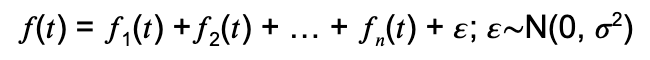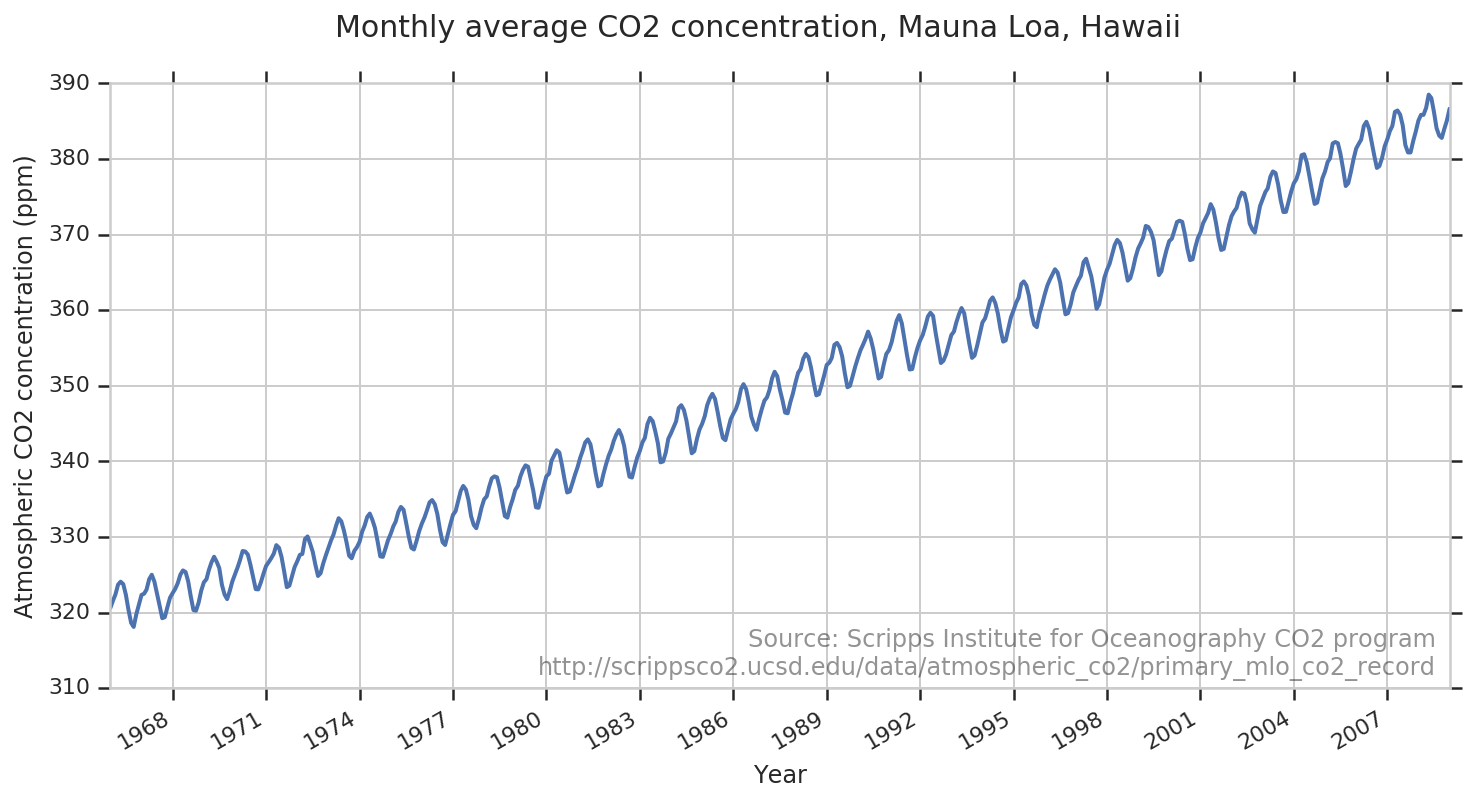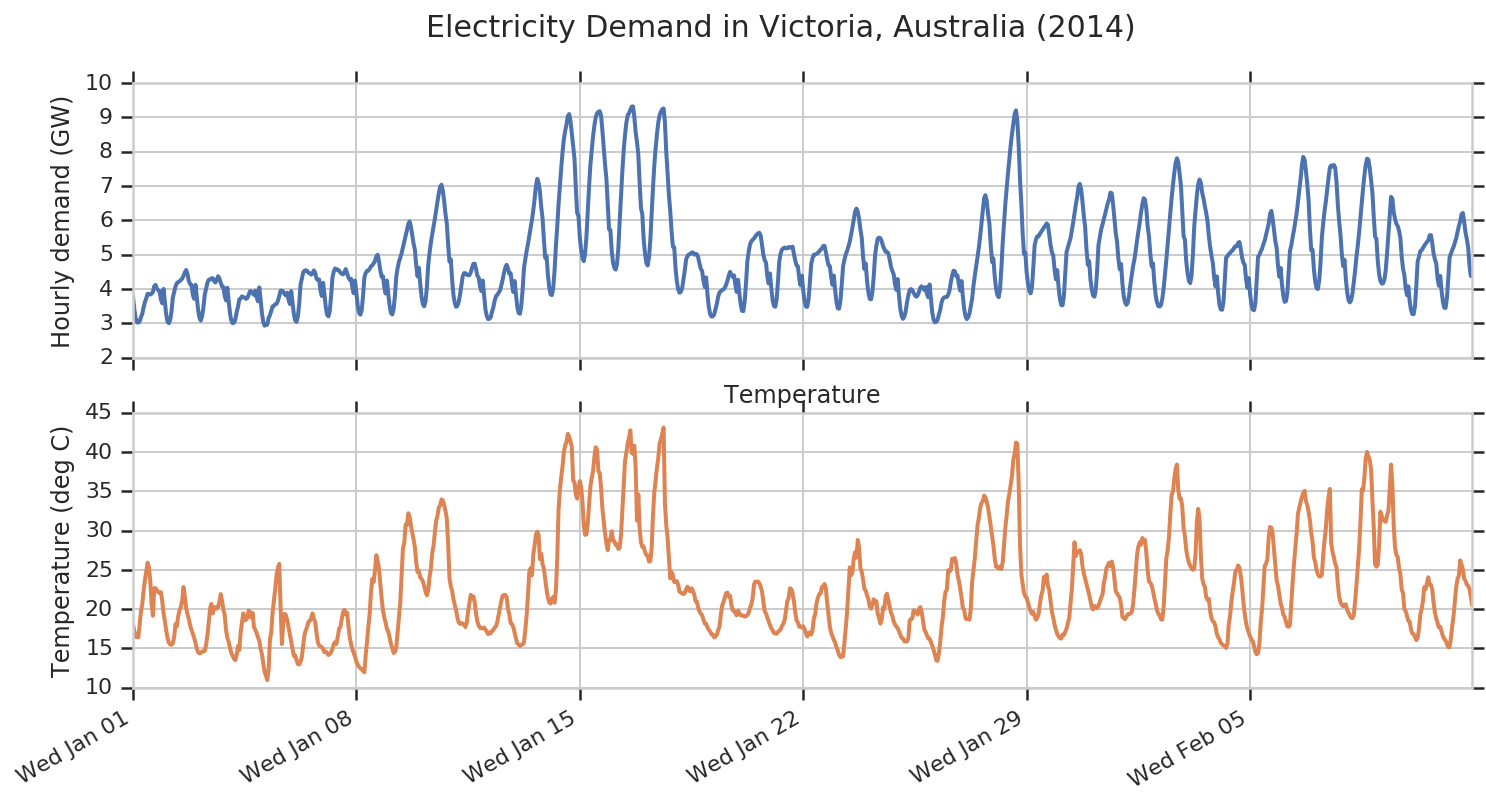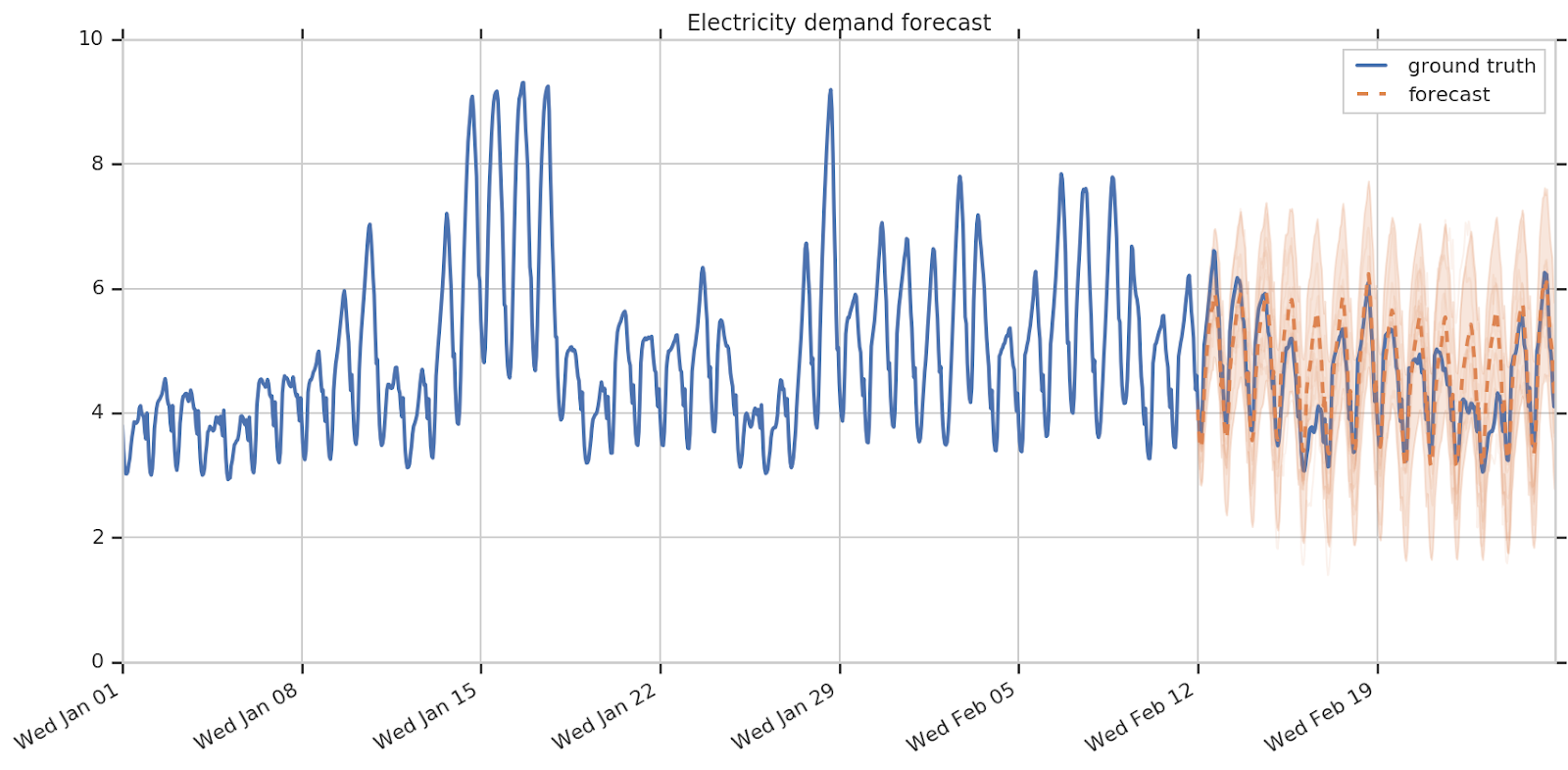TensorFlow Probability ·Structural Time Series modeling in TensorFlow Probability
اسفند ۲۹, ۱۳۹۷
Posted by Dave Moore, Jacob Burnim, and the TFP Team

In this post, we introduce `tfp.sts`, a new library in TensorFlow Probability for forecasting time series using structural time series models .

### Overview

“It is difficult to make predictions, especially about the future.”
Karl Kristian Steincke

Although predictions of future events are necessarily uncertain, forecasting is a critical part of planning for the future. Website owners need to forecast the number of visitors to their site in order to provision sufficient hardware resources, as well as predict future revenue and costs. Businesses need to forecast future demands for consumer products to maintain sufficient inventory of their products. Power companies need to forecast demand for electricity, to make informed purchases of energy contracts and to construct new power plants.

Methods for forecasting time series can also be applied to infer the causal impact of a feature launch or other intervention on user engagement metrics , to infer the current value of difficult-to-observe quantities like the unemployment rate from more readily available information , as well as to detect anomalies in time series data.

### Structural Time Series

Structural time series (STS) models  are a family of probability models for time series that includes and generalizes many standard time-series modeling ideas, including:

• autoregressive processes,
• moving averages,
• local linear trends,
• seasonality, and
• regression and variable selection on external covariates (other time series potentially related to the series of interest).

An STS model expresses an observed time series as the sum of simpler components:The individual components are each time series governed by a particular structural assumption. For example, one component might encode a seasonal effect (e.g., day-of-week effects), another a local linear trend, and another a linear dependence on some set of covariate time series.

By allowing modelers to encode assumptions about the processes generating the data, structural time series can often produce reasonable forecasts from relatively little data (e.g., just a single input series with tens of points). The model’s assumptions are interpretable, and we can interpret the predictions by visualizing the decompositions of past data and future forecasts into structural components. Moreover, structural time series models use a probabilistic formulation that can naturally handle missing data and provide a principled quantification of uncertainty.

### Structural Time Series in TensorFlow Probability

TensorFlow Probability (TFP) now features built-in support for fitting and forecasting using structural time series models. This support includes Bayesian inference of model parameters using variational inference (VI) and Hamiltonian Monte Carlo (HMC), computing both point forecasts and predictive uncertainties. Because they’re built in TensorFlow, these methods naturally take advantage of vectorized hardware (GPUs and TPUs), can efficiently process many time series in parallel, and can be integrated with deep neural networks.

### Example: Forecasting CO2 Concentration

To see structural time series in action, consider this monthly record of atmospheric CO2 concentration from the Mauna Loa observatory in Hawaii :It should be clear by inspection that this series contains both a long-term trend and annual seasonal variation. We can encode these two components directly in a structural time series model, using just a few lines of TFP code:
``````import tensorflow_probability as tfp
trend = tfp.sts.LocalLinearTrend(observed_time_series=co2_by_month)
seasonal = tfp.sts.Seasonal(
num_seasons=12, observed_time_series=co2_by_month)
model = tfp.sts.Sum([trend, seasonal], observed_time_series=co2_by_month)``````
Here we’ve used a local linear trend model, which assumes the trend is linear, with slope evolving slowly over time following a random walk. Fitting the model to the data produces a probabilistic forecast based on our modeling assumptions:We can see that the forecast uncertainty (shading ± 2 standard deviations) increases over time, as the linear trend model becomes less confident in its extrapolation of the slope. The mean forecast combines the seasonal variational with a linear extrapolation of the existing trend, which appears to slightly underestimate the accelerating growth in atmospheric CO2, but the true values are still within the 95% predictive interval.

The full code for this example is available on Github.

### Example: Forecasting Demand for Electricity

Next we’ll consider a more complex example: forecasting electricity demand in Victoria, Australia. The top line of this plot shows an hourly record from the first six weeks of 2014 (data from , available at https://github.com/robjhyndman/fpp2-package):Here we have access to an external source of information: the temperature, which correlates with electrical demand for air conditioning. Remember that January is summer in Australia! Let’s incorporate this temperature data in a STS model, which can include external covariates via linear regression:
``````  temperature_effect = tfp.sts.LinearRegression(
design_matrix=tf.reshape(temperature - np.mean(temperature),
(-1, 1)), name='temperature_effect')
hour_of_day_effect = tfp.sts.Seasonal(
num_seasons=24,
observed_time_series=demand,
name='hour_of_day_effect')
day_of_week_effect = tfp.sts.Seasonal(
num_seasons=7,
num_steps_per_season=24,
observed_time_series=demand,
name='day_of_week_effect')
residual_level = tfp.sts.Autoregressive(
order=1,
observed_time_series=demand, name='residual')
model = tfp.sts.Sum([temperature_effect,
hour_of_day_effect,
day_of_week_effect,
residual_level],
observed_time_series=demand)``````
Note that we’ve also included multiple seasonality effects: an hour-of-day, a day-of-week effect, and an autoregressive component to model any unexplained residual effects. We could have used a simple random walk, but chose an autoregressive component because it maintains bounded variance over time.The forecast from this model isn’t perfect — there are apparently still some unmodeled sources of variation — but it’s not crazy, and again the uncertainties look reasonable. We can better understand this forecast by visualizing the decomposition into components (note that each component plot has a different y-axis scale):We see that the model has quite reasonably identified a large hour-of-day effect and a much smaller day-of-week effect (the lowest demand appears to occur on Saturdays and Sundays), as well as a sizable effect from temperature, and that it produces relatively confident forecasts of these effects. Most of the predictive uncertainty comes from the autoregressive process, based on its estimate of the unmodeled (residual) variation in the observed series.

A modeler might use this decomposition to understand how to improve the model. For example, they might notice that some spikes in temperature still seem to coincide with spikes in the AR residual, indicating that additional features or data transformations might help better capture the temperature effect.

The full code for this example is available on Github.

### The TensorFlow Probability STS Library

As the above examples show, STS models in TFP are built by adding together model components. STS provides modeling components like:

• Autoregressive, LocalLinearTrend, SemiLocalLinearTread, and LocalLevel. For modeling time series with a level or slope that evolves according to a random walk or other process.
• Seasonal. For time series depending on seasonal factors, such as the hour of the day, the day of the week, or the month of the year.
• LinearRegression. For time series depending on additional, time-varying covariates. Regression components can also be used to encode holiday or other date-specific effects.

STS provides methods for fitting the resulting time series models with variational inference and Hamiltonian Monte Carlo.

Check out our code, documentation, and further examples on the TFP home page.

Structural time series are being used for several important time series applications inside Google. We hope you will find them useful, as well. Please join the tfprobability@tensorflow.org forum for the latest Tensorflow Probability announcements and other TFP discussions!

### References

 Brodersen, K. H., Gallusser, F., Koehler, J., Remy, N., & Scott, S. L. (2015). Inferring causal impact using Bayesian structural time-series models. The Annals of Applied Statistics, 9(1), 247–274.

 Choi, H., & Varian, H. (2012). Predicting the present with Google Trends. Economic Record, 88, 2–9.

 Harvey, A. C. (1989). Forecasting, structural time series models and the Kalman filter. Cambridge University Press.

 Hyndman, R.J., & Athanasopoulos, G. (2018). Forecasting: principles and practice, 2nd edition, OTexts: Melbourne, Australia. OTexts.com/fpp2. Accessed on February 23, 2019.

 Keeling, C. D., Piper, S. C., Bacastow, R. B., Wahlen, M., Whorf, T. P., Heimann, M., & Meijer, H. A. (2001). Exchanges of atmospheric CO2 and 13CO2 with the terrestrial biosphere and oceans from 1978 to 2000. I. Global aspects, SIO Reference Series, №01–06, Scripps Institution of Oceanography, San Diego.
Next postTensorFlow Probability ·Posted by Dave Moore, Jacob Burnim, and the TFP Team

In this post, we introduce tfp.sts, a new library in TensorFlow Probability for forecasting time series using structural time series models .
Overview“It is difficult to make predictions, especially about the future.”
Karl Kristian Steincke

Although predictions of future events are necessarily uncertain, forecasting is a critical part of pl…